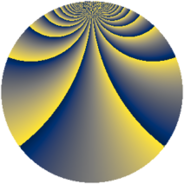# Properties

 Label 1425.2.clLevel $1425$ Weight $2$ Character orbit 1425.cl Rep. character $\chi_{1425}(41,\cdot)$ Character field $\Q(\zeta_{90})$ Dimension $4704$ Sturm bound $400$

# Related objects

## Defining parameters

 Level: $$N$$ $$=$$ $$1425 = 3 \cdot 5^{2} \cdot 19$$ Weight: $$k$$ $$=$$ $$2$$ Character orbit: $$[\chi]$$ $$=$$ 1425.cl (of order $$90$$ and degree $$24$$) Character conductor: $$\operatorname{cond}(\chi)$$ $$=$$ $$1425$$ Character field: $$\Q(\zeta_{90})$$ Sturm bound: $$400$$

## Dimensions

The following table gives the dimensions of various subspaces of $$M_{2}(1425, [\chi])$$.

Total New Old
Modular forms 4896 4896 0
Cusp forms 4704 4704 0
Eisenstein series 192 192 0

## Trace form

 $$4704q - 18q^{3} - 36q^{4} - 18q^{6} - 48q^{7} - 18q^{9} + O(q^{10})$$ $$4704q - 18q^{3} - 36q^{4} - 18q^{6} - 48q^{7} - 18q^{9} - 48q^{10} - 27q^{12} - 36q^{13} - 81q^{15} - 60q^{16} - 36q^{19} + 9q^{21} + 24q^{22} - 36q^{24} - 12q^{25} - 27q^{27} + 36q^{28} - 33q^{30} - 54q^{31} - 72q^{33} - 36q^{34} - 54q^{36} - 36q^{39} - 102q^{40} + 36q^{42} - 84q^{43} + 69q^{45} - 162q^{46} - 114q^{48} - 2064q^{49} + 12q^{51} - 72q^{52} + 6q^{54} + 30q^{55} + 114q^{57} + 144q^{58} + 141q^{60} - 108q^{61} + 117q^{63} + 486q^{64} + 114q^{66} - 132q^{67} - 27q^{69} + 204q^{70} - 120q^{72} - 36q^{73} - 96q^{76} - 117q^{78} - 36q^{79} + 30q^{81} - 384q^{82} + 297q^{84} + 54q^{85} - 57q^{87} - 54q^{88} - 174q^{90} + 90q^{91} - 234q^{93} - 132q^{96} - 60q^{97} - 90q^{99} + O(q^{100})$$

## Decomposition of $$S_{2}^{\mathrm{new}}(1425, [\chi])$$ into newform subspaces

The newforms in this space have not yet been added to the LMFDB.